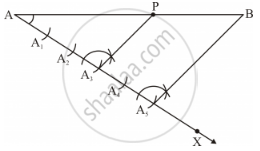Share

# Draw a Line Segment Ab of Length 7 Cm. Using Ruler and Compasses, Find a Point P on Ab Such that a P a B = 3 5 . - Mathematics

Course

#### Question

Draw a line segment AB of length 7 cm. Using ruler and compasses, find a point P on AB such that (AP)/(AB)=3/5.

#### Solution

It is given that(AP)/(AB)=3/5.

therefore (AP)/(PB)=(AP)/(AB-AP)=3/(5-3)=3/2

Thus, point P divides line segment AB in the ratio 3: 2.

To draw a line segment AB of length 7 cm and mark a point P (using ruler and compass) such that (AP)/(PB)=3/5 i,e, (AP)/(PB)=3/2, the following steps are to be followed:

Step 1: Draw line segment AB of length 7 cm and draw a ray AX making an acute angle with line segment AB.

Step 2: Locate 5 (2 + 3) points i.e., A1, A2, A3, A4 and A5 on AX such that AA1 = A1 A2 = A2 A3 and so on.

Step 3: Join BA5.

Step 4: Through point A3 , draw a line parallel to BA5 (by making an angle equal to ∠AA5 B) at A3 intersecting AB at point P.Now, P is the required point on line segment AB of length 7 cm. This point satisfies the condition(AP)/(AB)=3/5.

Is there an error in this question or solution?